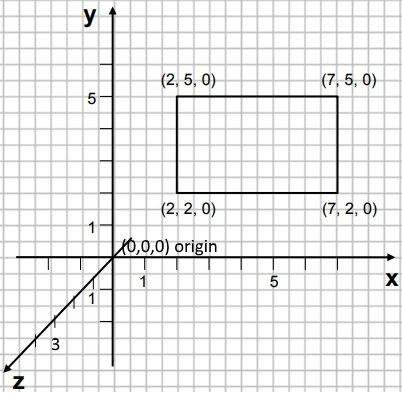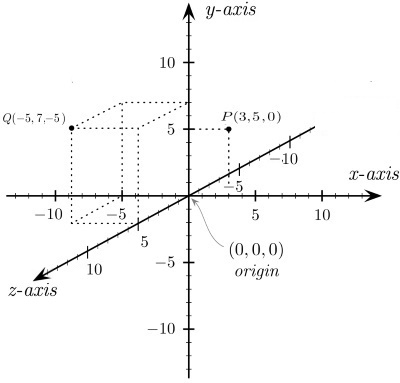#XDdom 3D Axis and Coordinate System by Stephen Bucaro

In order to code X3D graphics, you need to be able to define points in space. For this you need a 3D coordinate system. The 17th century mathematician Rene Descartes invented the Cartesian coordinate system. A coordinate system can be visualized as a 3D matrix in space. The coordinate system has three axis named x, y, and z.

The x-axis goes from left to right horizontally, the y-axis goes from bottom to top vertically, the z-axis goes from back to from outward towards the viewer. The center of the Cartesian coordinate system, called the origin, is a point in space where the value of all three coordinates is zero. A coordinate point at the origin would be x=0, y=0, z=0 or just 0,0,0. When specifying X3D coordinates, we often leave the commons between the x y z coordinates out e.g. 0 0 0.On the diagram above, the coordinates for the rectangle are "2 5 0, 7 5 0, 2 2 0, 7 2 0". Here we place commas between the coordinate points. In fact, in X3D we can often leave the commas between the coordinate points out because X3D already knows that every three digits defines a coordinate point.On the diagram above, the coordinates for the rectangular prism are:

"-5 7 5, -5 7 0, 0 7 0, 0 0 0,
-5 7 5, -5 7 0, -5 0 0, -5 0 -5,
0 7 5, 0 7 0, 0 0 0, 0 0 5,
-5 0 0, -5 7 0, 0 7 0, 0 0 0,
-5 7 5, 0 7 5, 0 0 5, -5 0 5"

Determining the 3D coordinates for a point while looking at a 2D plane is a tricky, but with practice it should become a bit easier.